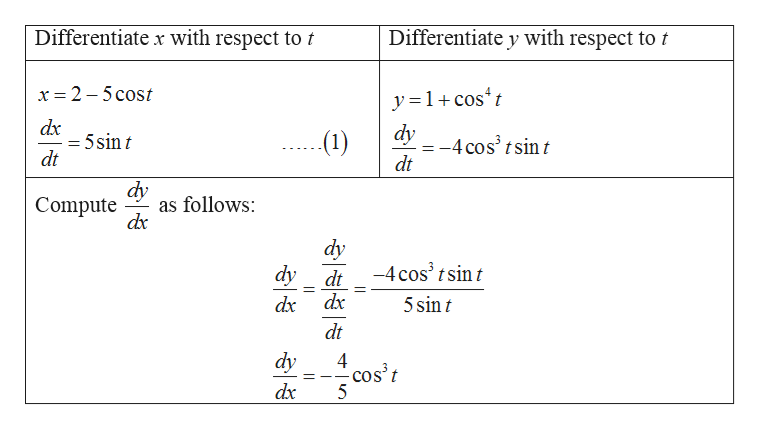Find d^2y/dx^2 as a function of t, for the given  parameteric equations written below show all workx = 2-5cos(t)y = 1 + cos^4(t)

Question

Find d^2y/dx^2 as a function of t, for the given  parameteric equations written below show all work

x = 2-5cos(t)

y = 1 + cos^4(t)

Step 1

From the parametric equation, the derivative...help_outlineImage TranscriptioncloseDifferentiate x with respect to t Differentiate y with respect to t x 2 5 cost y=1cost dx = 5sin t dt dy (1) =-4 cos3 tsint dt dy as follows dr Compute dy -4 cos3 t sin t dy dt dx dx 5 sin t dt dy 4 cos't 5 dx fullscreen

Want to see the full answer?

See Solution

Want to see this answer and more?

Our solutions are written by experts, many with advanced degrees, and available 24/7

See Solution
Tagged in

Derivative